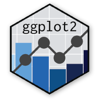Show Sidebar Hide Sidebar# geom_density2d in ggplot2

How to make a density map using geom_density2d.

### New to Plotly?

Plotly's R library is free and open source!
You can set up Plotly to work in online or offline mode.
We also have a quick-reference cheatsheet (new!) to help you get started!

### Version Check

Version 4 of Plotly's R package is now available!
Check out this post for more information on breaking changes and new features available in this version.

library(plotly)
packageVersion('plotly')

##  '4.9.1'


### Basic 2D Graph

library(plotly)
beers <- read.csv("https://raw.githubusercontent.com/plotly/datasets/master/beers.csv", stringsAsFactors = FALSE)

p <- ggplot(beers, aes(x=abv, y=ibu)) +
geom_density2d() +
labs(y = "bitterness (IBU)",
x = "alcohol volume (ABV)",
title = "Craft beers from American breweries")

ggplotly(p)


### Filled

Since each of the lines (in the above graph) shows a different "level", setting "fill = stat(level)" allows for a filled graph.

library(plotly)
beers <- read.csv("https://raw.githubusercontent.com/plotly/datasets/master/beers.csv", stringsAsFactors = FALSE)

p <- ggplot(beers, aes(x=abv, y=ibu)) +
stat_density2d(aes(fill = stat(level)), geom="polygon") +
labs(y = "bitterness (IBU)",
x = "alcohol volume (ABV)",
title = "Craft beers from American breweries")

ggplotly(p)


### Preset Colourscale

"Viridis" colourscales are designed to still be perceptible in black-and-white, as well as for those with colourblindness. It comes with five colourscales, selected using the option= parameter: "magma" (or "A"), "inferno" (or "B"), "plasma" (or "C"), "viridis" (or "D", the default), and "cividis" (or "E").

library(plotly)
beers <- read.csv("https://raw.githubusercontent.com/plotly/datasets/master/beers.csv", stringsAsFactors = FALSE)

p <- ggplot(beers, aes(x=abv, y=ibu)) +
stat_density2d(aes(fill = stat(level)), geom="polygon") +
scale_fill_viridis_c(option = "plasma") +
theme(legend.position = "magma") +
labs(y = "bitterness (IBU)",
x = "alcohol volume (ABV)",
title = "Craft beers from American breweries")

ggplotly(p)


### Customized Colourscale

You can also set your own colour gradients by defining a high and low point.

library(plotly)
beers <- read.csv("https://raw.githubusercontent.com/plotly/datasets/master/beers.csv", stringsAsFactors = FALSE)

p <- ggplot(beers, aes(x=abv, y=ibu)) +
stat_density2d(aes(fill = stat(level)), geom="polygon") +
scale_fill_gradient(low = "lightskyblue1", high = "darkred") +
theme(legend.position = "none") +
labs(y = "bitterness (IBU)",
x = "alcohol volume (ABV)",
title = "Craft beers from American breweries")

ggplotly(p)


### Overlaid Points

I use variable "style2" to filter out the six most common beer styles. This way, we can see that the cluster of beers in the top right (i.e. more bitter and higher alcohol content) are IPAs - perhaps unsurprisingly.

library(plotly)
library(dplyr)
beers <- read.csv("https://raw.githubusercontent.com/plotly/datasets/master/beers.csv", stringsAsFactors = FALSE)

p <- ggplot(beers, aes(x=abv, y=ibu)) +
geom_density2d(alpha=0.5) +
geom_point(data=filter(beers, !is.na(style2)), aes(colour=style2, text = label), alpha=0.3) +
labs(y = "bitterness (IBU)",
x = "alcohol volume (ABV)",
title = "Craft beers from American breweries",
colour = "Beer types")

ggplotly(p)